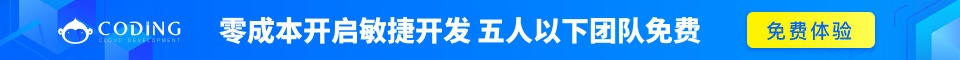# Python log() 函数Python 数字

## 描述

log() 方法返回x的自然对数。

## 语法

```import math

math.log( x )
```

• x -- 数值表达式。

## 实例

```#!/usr/bin/python
import math   # This will import math module

print "math.log(100.12) : ", math.log(100.12)
print "math.log(100.72) : ", math.log(100.72)
print "math.log(119L) : ", math.log(119L)
print "math.log(math.pi) : ", math.log(math.pi)
```

```math.log(100.12) :  4.60636946656
math.log(100.72) :  4.61234438974
math.log(119L) :  4.77912349311
math.log(math.pi) :  1.14472988585
```Python 数字